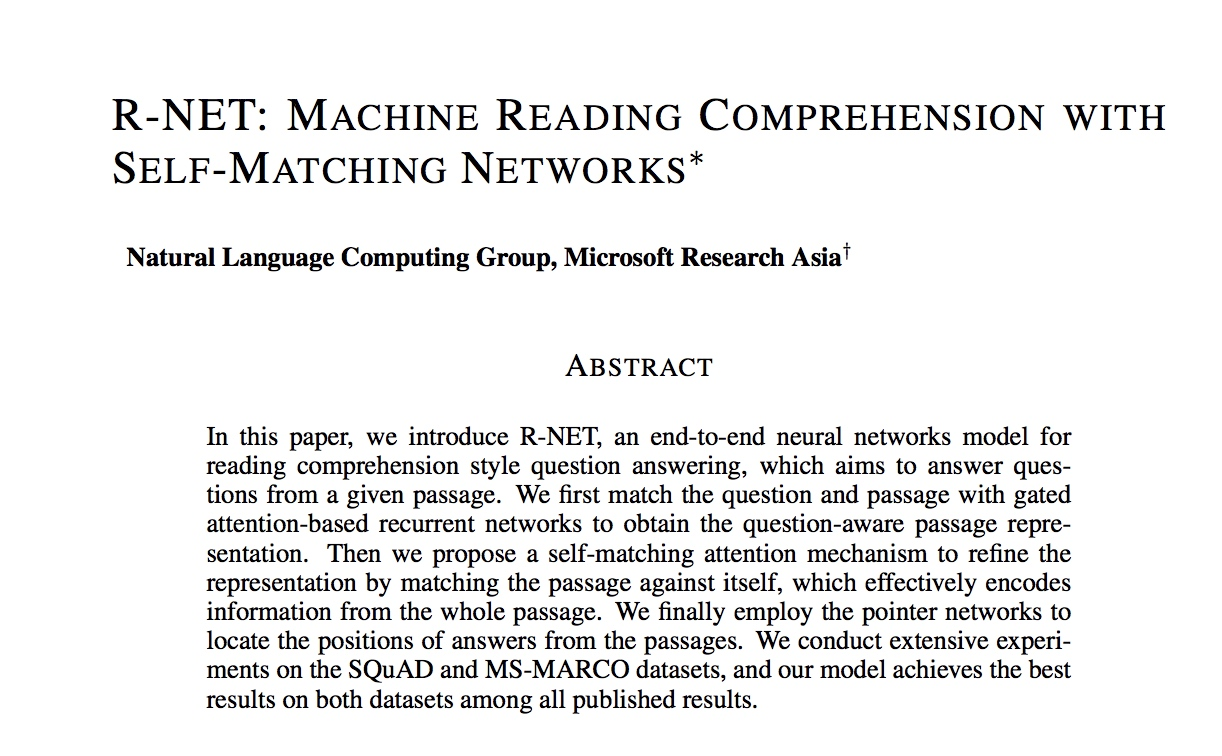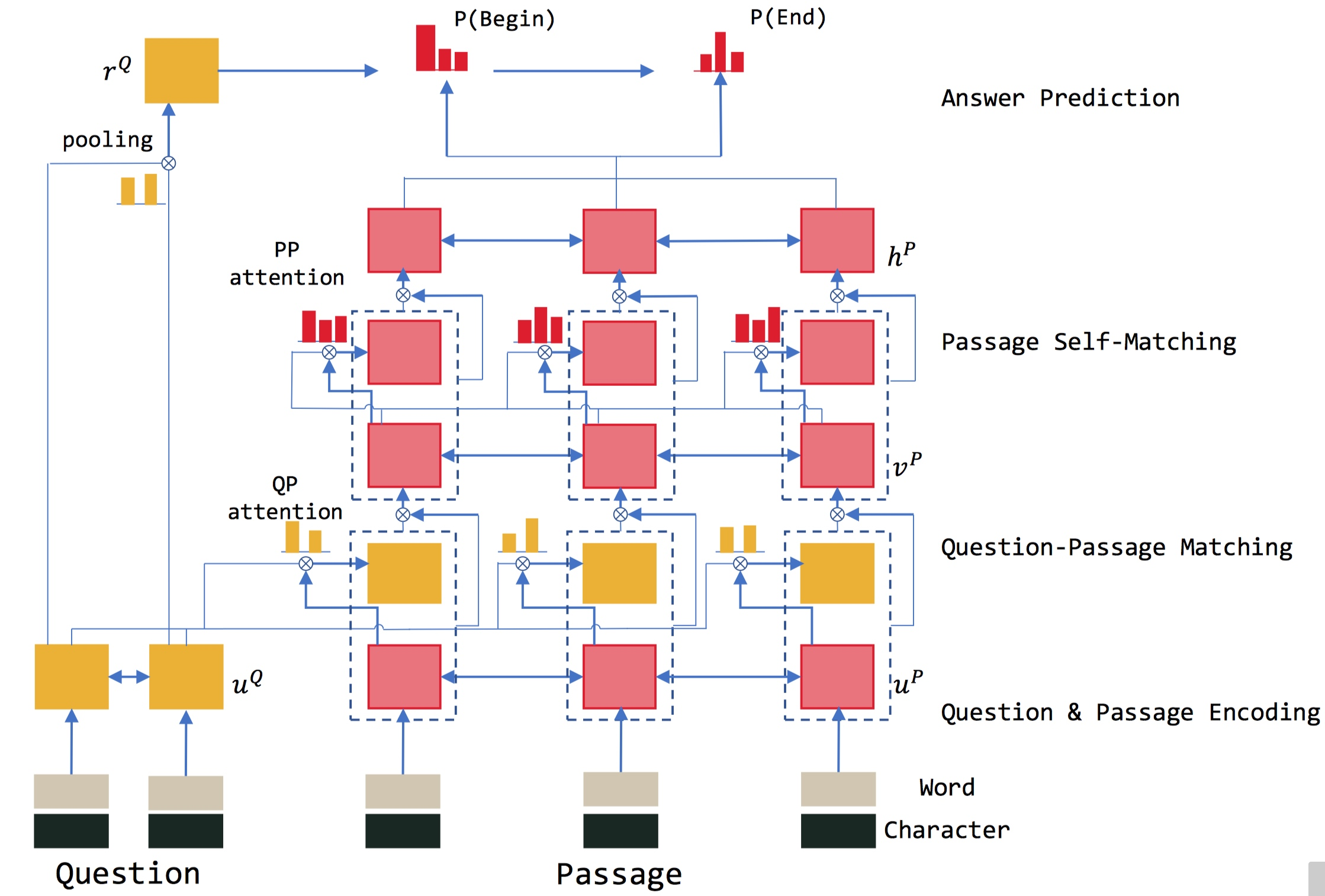# 用 R-Net Plus 来做阅读理解任务（上）

• 机器学习基础知识
• 一部分的统计学理论
• 模型复现经验
• 任务分析能力

## 开始## 论文的结构

R-NET 模型一共分为四个步骤，分别为：QUESTION AND PASSAGE ENCODER, GATED ATTENTION-BASED RECURRENT NETWORKS, SELF-MATCHING ATTENTION, OUTPUT LAYER。

### GATED ATTENTION-BASED RECURRENT NETWORKS

$$a_{i}^{t}=exp\left ( s_{i}^{t} \right )/\sum {j=1}^{m}exp\left ( s{j}^{t} \right)$$

$$c_{t}=\sum {i=1}^{m}a{i}^{t}u_{i}^{Q}$$

R-NET 又基于 matching-LSTM 和门控制方式对以上的方式提出了两点改进。第一点是把并联为[ , ]加入 RNN 中。即

## 参考链接/文章

各种Vec的不同作用
R-NET 阅读理解解析

# Bon
###### Your browser is out-of-date!

Update your browser to view this website correctly. Update my browser now

×